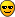# Perl Structure Conversion

pack(TEMPLATE,LIST)
Takes an array or list of values and packs it into a binary structure, returning the string containing the structure. The TEMPLATE is a sequence of characters that give the order and type of values, as follows:

A An ascii string, will be space padded.
a An ascii string, will be null padded.
c A signed char value.
C An unsigned char value.
s A signed short value.
S An unsigned short value.
i A signed integer value.
I An unsigned integer value.
l A signed long value.
L An unsigned long value.
n A short in `network' order.
N A long in `network' order.
f A single-precision float in the native format.
d A double-precision float in the native format.
p A pointer to a string.
v A short in `VAX' (little-endian) order.
V A long in `VAX' (little-endian) order.
x A null byte.
X Back up a byte.
@ Null fill to absolute position.
u A uuencoded string.
b A bit string (ascending bit order, like vec()).
B A bit string (descending bit order).
h A hex string (low nybble first).
H A hex string (high nybble first).

Each letter may optionally be followed by a number which gives a repeat count. With all types except `a', `A', `b', `B', `h', and `H', the pack function will gobble up that many values from the LIST. A `*' for the repeat count means to use however many items are left. The `a' and `A' types gobble just one value, but pack it as a string of length count, padding with nulls or spaces as necessary. (When unpacking, `A' strips trailing spaces and nulls, but `a' does not.) Likewise, the `b' and `B' fields pack a string that many bits long. The `h' and `H' fields pack a string that many nybbles long. Real numbers (floats and doubles) are in the native machine format only; due to the multiplicity of floating formats around, and the lack of a standard "network" representation, no facility for interchange has been made. This means that packed floating point data written on one machine may not be readable on another - even if both use IEEE floating point arithmetic (as the endian-ness of the memory representation is not part of the IEEE spec). Note that perl uses doubles internally for all numeric calculation, and converting from double to float back to double will lose precision (i.e. `unpack("f", pack("f", \$foo))' will not in general equal `\$foo'). Examples:

\$foo = pack("cccc",65,66,67,6;
# foo eq "ABCD"
\$foo = pack("c4",65,66,67,6;
# same thing

\$foo = pack("ccxxcc",65,66,67,6;
# foo eq "AB\0\0CD"

\$foo = pack("s2",1,2);
# "\1\0\2\0" on little-endian
# "\0\1\0\2" on big-endian

\$foo = pack("a4","abcd","x","y","z");
# "abcd"

\$foo = pack("aaaa","abcd","x","y","z");
# "axyz"

\$foo = pack("a14","abcdefg");
# "abcdefg\0\0\0\0\0\0\0"

\$foo = pack("i9pl", gmtime);
# a real struct tm (on my system anyway)

sub bintodec {
unpack("N", pack("B32", substr("0" x 32 . shift, -32)));
}

The same template may generally also be used in the unpack function.
unpack(TEMPLATE,EXPR)
unpack does the reverse of pack: it takes a string representing a structure and expands it out into an array value, returning the array value. (In a scalar context, it merely returns the first value produced.) The TEMPLATE has the same format as in the pack function. Here's a subroutine that does substring:

sub substr {
local(\$what,\$where,\$howmuch) = @_;
unpack("x\$where a\$howmuch", \$what);
}

and then there's

sub ord { unpack("c",\$_); }

In addition, you may prefix a field with a `%' to indicate that you want a -bit checksum of the items instead of the items themselves. Default is a 16-bit checksum. For example, the following computes the same number as the System V sum program:

while (<>) {
\$checksum += unpack("%16C*", \$_);
}
\$checksum %= 65536;